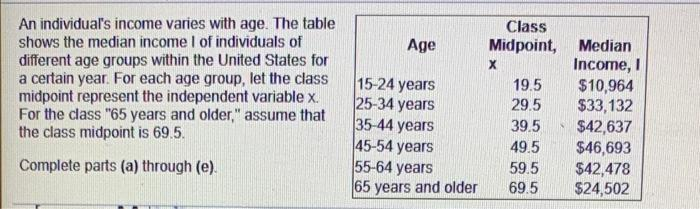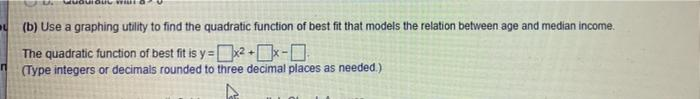# Question Solved1 AnswerAge An individual's income varies with age. The table shows the median income of individuals of different age groups within the United States for a certain year. For each age group, let the class midpoint represent the independent variable x. For the class "65 years and older," assume that the class midpoint is 69.5. 15-24 years 25-34 years 35 44 years 45-54 years 55-64 years Class Midpoint, Median х Income, 1 19.5 $10,964 29.5$33,132 39.5 $42,637 49.5$46,693 59.5 $42,478 69.5$24,502 Complete parts (a) through (e) 65 years and older (b) Use a graphing utility to find the quadratic function of best fit that models the relation between age and median Income. The quadratic function of best fit is y=x2+Ix- (Type integers or decimals rounded to three decimal places as needed.) ПTranscribed Image Text: Age An individual's income varies with age. The table shows the median income of individuals of different age groups within the United States for a certain year. For each age group, let the class midpoint represent the independent variable x. For the class "65 years and older," assume that the class midpoint is 69.5. 15-24 years 25-34 years 35 44 years 45-54 years 55-64 years Class Midpoint, Median х Income, 1 19.5 $10,964 29.5$33,132 39.5 $42,637 49.5$46,693 59.5 $42,478 69.5$24,502 Complete parts (a) through (e) 65 years and older (b) Use a graphing utility to find the quadratic function of best fit that models the relation between age and median Income. The quadratic function of best fit is y=x2+Ix- (Type integers or decimals rounded to three decimal places as needed.) П
More
Transcribed Image Text: Age An individual's income varies with age. The table shows the median income of individuals of different age groups within the United States for a certain year. For each age group, let the class midpoint represent the independent variable x. For the class "65 years and older," assume that the class midpoint is 69.5. 15-24 years 25-34 years 35 44 years 45-54 years 55-64 years Class Midpoint, Median х Income, 1 19.5 $10,964 29.5$33,132 39.5 $42,637 49.5$46,693 59.5 $42,478 69.5$24,502 Complete parts (a) through (e) 65 years and older (b) Use a graphing utility to find the quadratic function of best fit that models the relation between age and median Income. The quadratic function of best fit is y=x2+Ix- (Type integers or decimals rounded to three decimal places as needed.) П